Courses

# Magnetic Effects Of Current MCQ Level - 1

## 25 Questions MCQ Test Exclusive Video Lectures of Class 12 Physics by Experts | Magnetic Effects Of Current MCQ Level - 1

Description
This mock test of Magnetic Effects Of Current MCQ Level - 1 for Class 12 helps you for every Class 12 entrance exam. This contains 25 Multiple Choice Questions for Class 12 Magnetic Effects Of Current MCQ Level - 1 (mcq) to study with solutions a complete question bank. The solved questions answers in this Magnetic Effects Of Current MCQ Level - 1 quiz give you a good mix of easy questions and tough questions. Class 12 students definitely take this Magnetic Effects Of Current MCQ Level - 1 exercise for a better result in the exam. You can find other Magnetic Effects Of Current MCQ Level - 1 extra questions, long questions & short questions for Class 12 on EduRev as well by searching above.
QUESTION: 1

Solution:
QUESTION: 2

Solution:
QUESTION: 3

### A conducting rod of mass m and length l is placedover a smooth horizontal surface. A uniform magneticfield B is acting perpendicular to the rod. Charge q issuddenly passed through the rod and it acquires aninitial velocity v on the surface, then q is equal to

Solution:
QUESTION: 4

A wire is wound on a long rod of material of relativepermeability μr = 4000 to make a solenoid. If the currentthrough the wire is 5 A and number of turns per unitlength is 1000 per metre, then the magnetic field insidethe solenoid is :

Solution:
QUESTION: 5

The magnetic field at the point of intersection of diagonals of a square loop of side L carrying a current I is

Solution:
QUESTION: 6

Two particles X and Y having equal charges, afterbeing accelerated through the same potential difference, enter a region of uniform magnetic fieldand describe circular path of radii R1 and R2 respectively. The ratio of mass of X to the mass Y is

Solution:
QUESTION: 7

Two identical coils carry equal currents and have acommon centre, but their planes are at right anglesto each other. What is the magnitude of the resultantmagnetic field at the centre if the field due to one coilalone is B?

Solution:
QUESTION: 8

A coaxial cable is made up of two conductors. Theinner conductor is solid and is of radius R1 & theouter conductor is hollow of inner radius R2 and outerradius R3. The space between the conductors is filledwith air. The inner and outer conductors are carryingcurrents of equal magnitudes and in oppositedirections. Then the variation of magnetic field withdistance from the axis is best plotted as: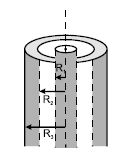Solution:
QUESTION: 9

Three long, straight and parallel wires C, D and Gcarrying currents are arranged as shown in the figure.The force experienced by a 25 cm length of wire C is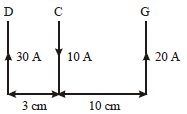Solution:
QUESTION: 10

A potential difference of 600 V is applied across theplates of a parallel plate capacitor. The separationbetween the plates is 3 mm. An electron projectedvertically, parallel to the plates, with a velocity of 2× 106 ms–1 moves undeflected between the plates.What is the magnitude of the magnetic field betweenthe capacitor plates?

Solution:
QUESTION: 11

Two particles each of mass m are charged q, areattached to the two ends of a light rigid rod of length 2l. The rod is rotated at a constant angular speed abouta perpendicular axis passing through its centre. Theratio of the magnitudes of the magnetic moment ofthe system and its angular momentum about the centreof the rod is

Solution:
QUESTION: 12

Axis of a solid cylinder of infinite length and radius R lies along y-axis it carries a uniformlydistributed current ‘ i ’ along  +y direction. Magnetic fieldat a point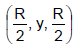is :-

Solution:
QUESTION: 13

Two very long straight parallel wires carry steadycurrents I and –I. The distance between the wires isd. At a certain instant of time, a point charge q is at apoint equidistant from the two wires, in the plane ofthe wires. Its instantaneous velocity v is perpendicularto this plane. The magnitude of the force due to themagnetic field acting on the charge at this instant is

Solution:
QUESTION: 14

A rectangular loop carrying a current i is situated neara long straight wire such that the wire is parallel tothe one of the sides of the loop and is in the plane ofthe loop. If a steady current I is established in wireas shown in figure, the loop will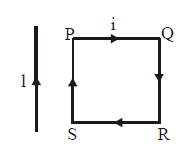Solution:
QUESTION: 15

A steady current I flows in a small square loop ofwire of side L in a horizontal plane. The loop is nowfolded about its middle such that half of it lies in avertical plane. Let μ1 and μ2 respectively denote themagnetic moments due to the current loop before andafter folding. Then

Solution:
QUESTION: 16

A cylindrical wire of radius R is carrying current iuniformly distributed over its cross-section. If a circular loop of radius ' r ' is taken as amperican loop,then the variation value of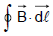over this loop withradius ' r ' of loop will be best represented by:

Solution:
QUESTION: 17

An insulating rod of length L carries a charge Qdistributed uniformly on it. The rod is pivoted at one end and is rotated at a frequency f and about a fixed perpendicular axis. The magnetic dipole moment of the system is

Solution:
QUESTION: 18

A wire of resistance R in the form of a semicircle lieson the top of a smooth table. A uniform magnetic field B is confined to the region as shown. The ends of the semicircle are attached to springs C and D whose other ends are fixed. If r is the radius of the semicircle and k is the force constant for each spring, then the extension x in each spring is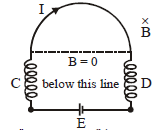Solution:
QUESTION: 19

A circular current loop of radius a is placed in ara dial field B as shown. The net force acting on the loop is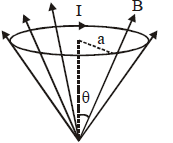Solution:
QUESTION: 20

Two parallel, long wires carry currents i1 and i2 with i1 >i2. When the current are in the same direction, the magnetic field at a point midway between the wire is10mT. If the direction of i2 is reversed, the field becomes 30μT. The ratio i1/i2 is

Solution:
QUESTION: 21

A positively charged particle projected towards east isdeflected towards north by a magnetic field. The fieldmay be

Solution:
QUESTION: 22

Which of the following particles will describe the smallestcircle when projected with the same velocity perpendicularto a magnetic field?

Solution:
QUESTION: 23

An electric current i enters and leaves a uniform circularwire of radius a through diametrically opposite points.A charged particle q moving along the axis of the circularwire passes through its centre at speed u. The magneticforce acting on the particle when it passes throughthe centre has a magnitude

Solution:
QUESTION: 24

A negative charged particle falling freely under gravityenters a region having uniform horizontal magnetic field pointing towards north. The particle willbe deflected towards

Solution:
QUESTION: 25

A proton of mass m and charge q enters a magneticfield B with a velocity v at an angle q with the directionof B. The radius of the resulting path is

Solution: